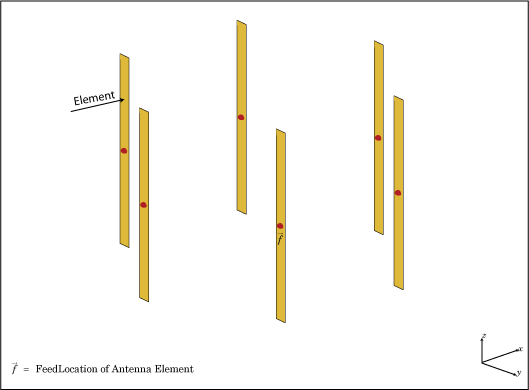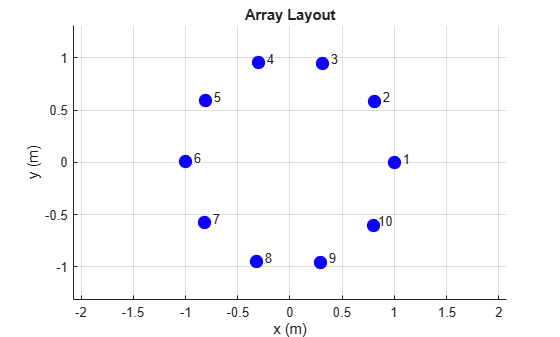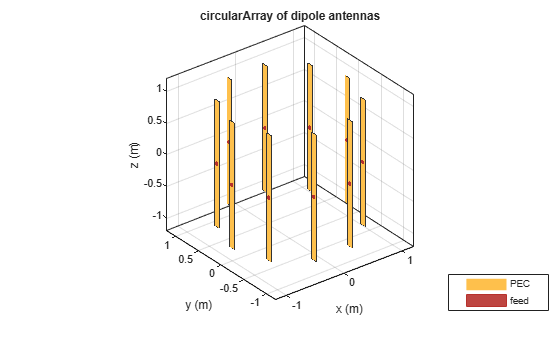# circularArray

Create circular antenna array

## Description

The `circularArray` object creates a circular antenna array. Circular array finds application in direction of arrival (DoA) systems. You can use circular arrays to perform 2-D scanning, while lowering element counts. These arrays also have the ability for 360-degree scanning. The individual elements in the circular array are part of the same array environment. This property reduces the impact of edge effects and other coupling variation.## Creation

### Syntax

``array = circularArray``
``array = circularArray(Name=Value)``

### Description

``` `array = circularArray` creates a circular antenna array in the xy-plane. ```

example

``` `array = circularArray(Name=Value)` creates a circular array, with additional Properties specified by one or more name-value arguments. `Name` is the property name and `Value` is the corresponding value. You can specify several name-value pair arguments in any order as `Name1=Value1`,`...`, `NameN=ValueN`. Properties that you do not specify retain their default values.```

## Properties

expand all

Individual antenna type, specified as a single antenna object for homogeneous array or a vector of antenna objects of same type with varying dimensions and load for heterogeneous array. The antenna object can be an element from the Antenna Catalog or an antenna without a substrate created using `customAntennaGeometry`, `customAntennaMesh`, `customAntennaStl`, or `customAntenna` objects.

Note

Following antennas are unsupported as an element:

Example: `dipole`

Example: `[monopole monopole(Height=2)]`

Example: `customAntenna`

Number of elements in the array,specified as a positive scalar integer. The elements in the array are arranged along the x-axis.

Example: `4`

Data Types: `double`

Radius of array, specified as a positive scalar integer in meters.

Example: `0.4`

Data Types: `double`

Starting angle offset for first element in array, specified as a real scalar in degrees.

Example: `8`

Data Types: `double`

Excitation amplitude for antenna elements in the array, specified as a real positive vector of size `'Element'`.

Example: `[0 1]`

Data Types: `double`

Phase shift for each element in the array, specified as a real vector of size `'Element'` in degrees.

Example: `[0 2]`

Data Types: `double`

Tilt angle of the array in degrees, specified as a scalar or vector. For more information, see Rotate Antennas and Arrays.

Example: `90`

Example: `Tilt=[90 90]`,`TiltAxis=[0 1 0;0 1 1]` tilts the array at 90 degrees about the two axes, defined by the vectors.

Data Types: `double`

Tilt axis of the array, specified as one of these values:

• Three-element vectors of Cartesian coordinates in meters. In this case, each vector starts at the origin and lies along the specified points on the x-, y-, and z-axes.

• Two points in space, specified as a 2-by-3 matrix corresponding to two three-element vectors of Cartesian coordinates. In this case, the array rotates around the line joining the two points.

• `"x"`, `"y"`, or `"z"` to describe a rotation about the x-, y-, or z-axis, respectively.

Example: `[0 1 0]`

Example: `[0 0 0;0 1 0]`

Example: `"Z"`

Data Types: `double` | `string`

## Object Functions

 `show` Display antenna, array structures, shapes, or platform `info` Display information about antenna or array `arrayFactor` Array factor in dB `beamwidth` Beamwidth of antenna `charge` Charge distribution on antenna or array surface `correlation` Correlation coefficient between two antennas in array `current` Current distribution on antenna or array surface `design` Design prototype antenna or arrays for resonance around specified frequency or create AI-based antenna from antenna catalog objects `efficiency` Radiation efficiency of antenna `EHfields` Electric and magnetic fields of antennas or embedded electric and magnetic fields of antenna element in arrays `impedance` Input impedance of antenna or scan impedance of array `layout` Display array or PCB stack layout `mesh` Mesh properties of metal, dielectric antenna, or array structure `optimize` Optimize antenna or array using SADEA optimizer `pattern` Plot radiation pattern and phase of antenna or array or embedded pattern of antenna element in array `patternAzimuth` Azimuth plane radiation pattern of antenna or array `patternElevation` Elevation plane radiation pattern of antenna or array `rcs` Calculate and plot radar cross section (RCS) of platform, antenna, or array `returnLoss` Return loss of antenna or scan return loss of array `sparameters` Calculate S-parameters for antennas and antenna arrays `vswr` Voltage standing wave ratio (VSWR) of antenna or array element

## Examples

collapse all

Create a circular antenna array using 10 antenna elements. View the layout of the antenna elements in the array.

`ca = circularArray(NumElements=10)`
```ca = circularArray with properties: Element: [1x1 dipole] NumElements: 10 Radius: 1 AngleOffset: 0 AmplitudeTaper: 1 PhaseShift: 0 Tilt: 0 TiltAxis: [1 0 0] ```
```figure layout(ca)```View the array.

```figure show(ca)```Plot the elevation pattern of the circular array at a frequency of 70 MHz.

```figure patternElevation(ca,70e6)```## Version History

Introduced in R2016b